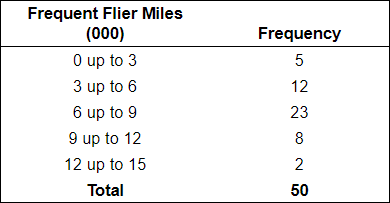×
Get Full Access to Basic Statistics For Business And Economics - 7 Edition - Chapter 2 - Problem 21e
Get Full Access to Basic Statistics For Business And Economics - 7 Edition - Chapter 2 - Problem 21e

×

The frequency distribution representing the number ofISBN: 9780077384470 64

Solution for problem 21E Chapter 2

Basic Statistics for Business and Economics | 7th Edition

• Textbook Solutions
• 2901 Step-by-step solutions solved by professors and subject experts
• Get 24/7 help from StudySoup virtual teaching assistantsBasic Statistics for Business and Economics | 7th Edition

4 5 1 357 Reviews
20
2
Problem 21E

The frequency distribution representing the number of frequent flier miles accumulated by employees at Brumley Statistical Consulting Company is repeated from Exercise 17.a.  How many employees accumulated less than 3,000 miles?

b.  Convert the frequency distribution to a cumulative frequency distribution.

c.  Portray the cumulative distribution in the form of a cumulative frequency polygon.

d.  Based on the cumulative frequency polygon, about 75 percent of the employees accumulated how many miles or less?

Step-by-Step Solution:

Step 1 of 3

The given frequency distribution representing the number of frequent flier miles accumulated by the employees in a company.

 Frequent Flier Miles (000) Frequency 0 up to 3 5 3 up to 6 12 6 up to 9 23 9 up to 12 8 12 up to 15 2 Total 50

By using the given information we have to find the following:

(a)  The number of employees accumulated less than 3,000 miles.

(b)  We have to convert the frequency distribution to a cumulative distribution.

(c)  We have to draw a cumulative frequency polygon.

(d)  Based on the cumulative frequency polygon, we have to find about 75 percent of the employee's accumulated how many miles or less.

Step 2 of 3

Step 3 of 3

ISBN: 9780077384470

Unlock Textbook Solution